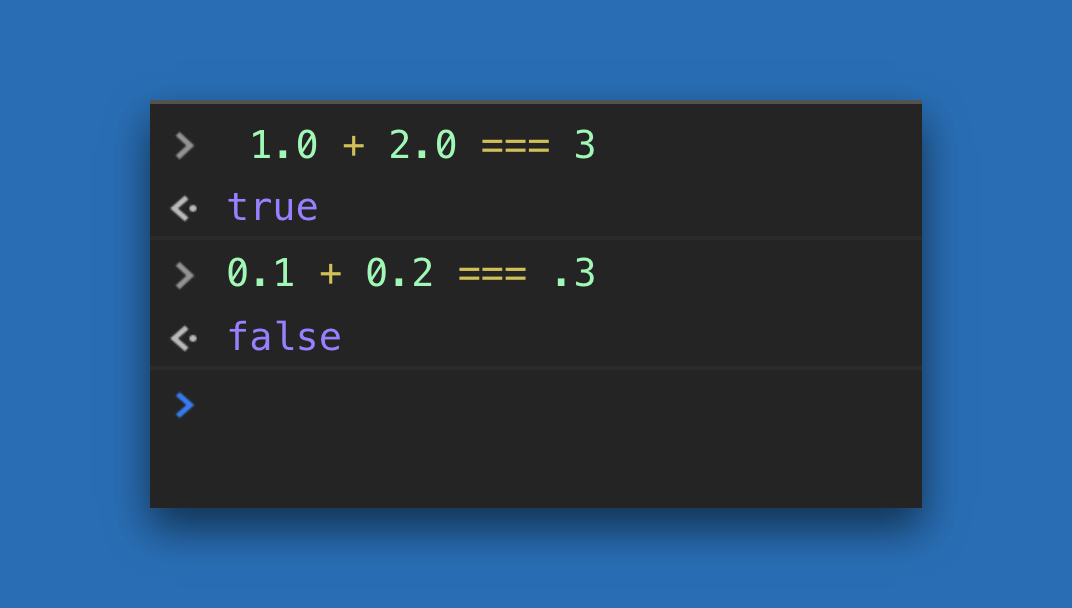# Money in Javascript

When accepting money online, it is important to be accurate. Just one cent off and your customers may lose trust in your application. That is why it is  It is important to understand how Javascript handles decimals.

By default, Javascript does not have a decimal datatype but it does have a Number type, which can be used as an integer or a double precision float. Because it’s a binary representation of a base 10 system, you end up with inaccurate results when you try to do the math.

So how do you get around these inconsistencies? The recommended approach is to store dollars in cents. Below are some helper functions I have used to convert between dollars and cents.

### Convert Dollars to Cents

```var formatDollarsToCents = function(value) {
value = (value + '').replace(/[^\d.-]/g, '');
if (value && value.includes('.')) {
value = value.substring(0, value.indexOf('.') + 3);
}

return value ? Math.round(parseFloat(value) * 100) : 0;
}```

### Convert Cents to Dollars

```var formatCentsToDollars = function(value) {
value = (value + '').replace(/[^\d.-]/g, '');
value = parseFloat(value);
return value ? value / 100 : 0;
}```

#### Key Takeaways

• Always store dollars in whole cents and display in dollars
• Use money javascript libraries to help with other currencies.
• Avoid .toFixed(2); because of rounding issues